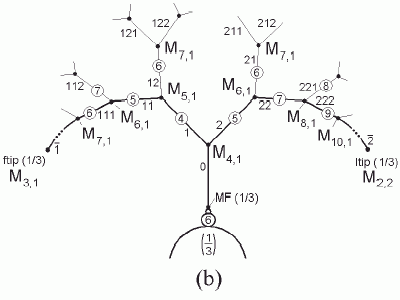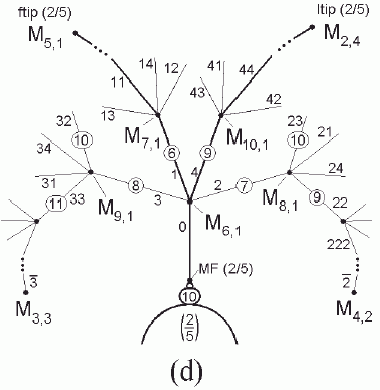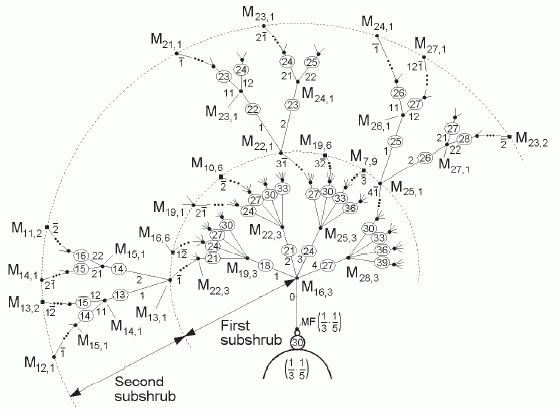Shrubs ordering
Miguel Romera

As can be seen in , the end of the period-doubling cascade of any hyperbolic component hc is its Myrberg-Feigenbaum point MF(hc). What emerges from MF(hc) is what we denominate the shrub of the hc, due to its shape. If the shrub emerges from a primary hc (p/q), then we have a primary shrub, the shrub(p/q). If the shrub emerges from a secondary, tertiary,..., N-ary hc, then we have a secondary, tertiary ,..., N-ary shrub. Secondary, tertiary, ..., N-ary shrubs have 2, 3, ..., N subshrubs. As can be seen in , we can calculate the period of the representative hyperbolic component rhc of each one of the structural branches of the shrubs, as well as the preperiod and period of each one of the Misiurewicz points from which structural branches emanate (nodes) or where the shrub branches end (tips).

Primary shrubs ordering

1. Shrub(1/3)

Shrub(1/3) is associated to a "decoration" formed by a node that is a Misiurewicz point from which three (p = 3) structural branches emanate. We can consider that the period-3 hyperbolic component 1/3, the origin of the shrub(1/3), is placed on the main branch 0, and that this branch 0 finishes on the main node 0, a Misiurewicz point M4,1. If we turn clockwise around the main node by following the branches 1 by 1 (because q = 1), we have the branches 0, 1 and 2 (or the hc's with periods 3, 4 and 5). At the end of each branch with a period-p rhc we have the Misiurewicz point Mp+1,1. So, at the end of the branch 1, whose rhc has period 4, we have the Misiurewicz point M5,1. If we turn clockwise around the M5,1 by following the branches 1 by 1, we have the hc's with periods 4, 5 and 6. Tips can also be found, being ftip(1/3) = M5,1. In such a manner we can find all the structural hc's and Misiurewicz points.2. Shrubs(2/5)Shrubs(2/5) is associated to a "decoration" formed by a node from which five (p = 5) structural branches emanate. If we turn clockwise around the main node (but now by following the branches 2 by 2! because here q = 2) we have the branches 0, 1, 2, 3 and 4 (or the hc's with periods 5, 6, 7, 8 and 9). Again, at the end of each branch with a period-p rhc we have the Misiurewicz point Mp+1,1. For example, at the end of the branch 2, whose rhc has period 7, we have the Misiurewicz point M8,1. If we turn clockwise around this M8,1 by following the branches 2 by 2, we have the hyperbolic components with periods 7, 8, 9, 10 and 11. Here flip(2/5) = M5,1. In such a manner we can find again all the structural hc's and Misiurewicz points.

Secondary shrubs ordering

Shrub(1/3 1/5) has two subshrubs, subshrub1(1/3 1/5) and subshrub2(1/3 1/5), that are associated to "decorations" formed by a node from which five (p2 = 5) and three (p1 = 3) structural branches emanate. In both subshrubs we turn clockwise around nodes by following the branches 1 by 1 because in this case q1 = q2 = 1. However, in the first subshrub periods increase 3 by 3 whilst in the second subshrub periods increase 1 by 1 . At the end of each branch of period p we have the Misiurewicz point Mp+1,3 in subshrub1(1/3 1/5) and Mp+1,1 in subshrub2(1/3 1/5). So, at the end of the branch 2 of subshrub1(1/3 1/5), whose rhc has period 21, we have the Misiurewicz point M22,3. If we turn clockwise around this M22,3 by following the branches 1 by 1, we have the hc's with periods 21, 24, 27, 30 and 33. Likewise, at the end of the branch 1- → 2 of the subshrub2(1/3 1/5), whose rhc has period 14, we have the Misiurewicz point M15,1. If we turn clockwise around the M15,1 by following the branches 1 by 1, we have the hc's with periods 14, 15 and 16. In such a manner we can find all the structural hc's and Misiurewicz points in both subshrubs. See  to calculate tips in both shrubs.Choose another shrub to "remove" labels.

 M.Romera, G.Pastor, G.Alvarez, and F.Montoya, "Shrubs in the Mandelbrot Set Ordering", accepted in International Journal of Bifucation and Chaos, 2002.

Contents   Previous: Embedded Julia midgets
updated 31 Dec 2013